## Tuesday, 6 December 2011

### Irrationality II

Last time we have discussed the basic features of irrational numbers and this time we may talk more about the relationship between irrationals and ploynomials.

A supplementary note on the irrationality of trigonometric functions

Recall that rational numbers can be expressed in forms of simpliest ratio between two integers, in which the function involved is division (related to multiplication which is the inverse of division), which implies that rational numbers form a group under multiplication. However irrational numbers can't form a group ifself.

Denote a polynomial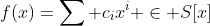if and only if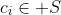. For example, for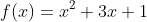,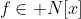. We call a polynomial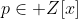a polynomial with integral coefficient, while calling a polynomial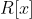a real polynomial. Our analysis prevent anything beyond real polynomial otherwise it will be far difficult.

Note that these symbols have their special meaning in group theory like the lattice point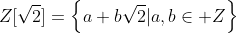, but this is boyond the scope of this passage.

Let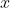be a irrational number, i.e.,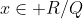and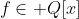. Then we say that if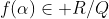, then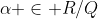. The inverse of this statement may NOT be true.
Proof:
Assume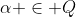, then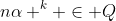where n is rational and k is non-negative integer, so as the concerned polynomial f. Therefore.
For example, we know that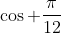is irrational sincewhile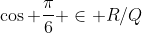. Simply putting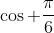into f gives the irrationality of the desired number.
However, the same arguement is not applicable on tangent function because it's angle sum formula is NOT a polynomial but a rational function.
We have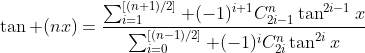. For example, we have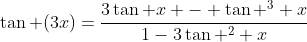. Although by the same arguement it works, but we shall prove the irrationality of tangent function by rewriting it into a continued fraction so that for every rational x, tan x is irrational unless it trivially is. (See Wolfram Mathworld)

An interesting fact is that the statement is not equivalent, however it CAN be equivalent. It should be easy for readers to find out its equivalent case when the inverse of f is considered, so it's proof  is left for readers.

Exercise. Find the necessary condition that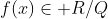is equivalent to.

We start from easier case: linear and quadratic functions.

Theorem. For linear, rationality of f(x) is equivalence to the rationality of x. Since rational number is a equivalence class, irrationality of f(x) also implies the irrationality of x.

Proof. If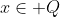, then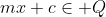for rational m and c. Inversely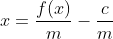which is also linear, so the rationality of x implies the rationality of f(x). For since the coefficients are rational, we can apply the same procedure to show the irratioanlity relation.

Theorem. For quadratic f, the statement is equivalent only when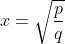, i.e., square root of a rational number.

Proof. left as exercise.

Sometimes problems concerning rational numbers are easier to be solved when rational numbers are replaced by integers. Then tools like congrunences can be applied easier.
For example, the statement "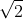is irrational" is equivalent to "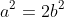is insoluble in integers" because you can assume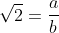, which is equivalent to the second statement. Similarly we can rewrite the statement "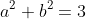is insoluble in rationals" (The method of Conic paramization) in equation over the integral domain. (Exercise)

The final aim of this passage is to proof the irrationality of more numbers.

Theorem.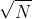is either integer (trivial case) or irrational.
Recall that it's equivalent to the statement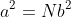is soluble in integers of simpliest ratio. It follows that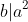and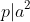for any prime factor p of b. Now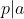so that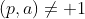which contradicts our assumption.

In fact this is a generalization of the second (historical) proof.

Here's another proof given by G.H. Hardy:
Assume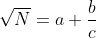where a,b,c are integers while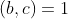with 0 < b < c. Nowso that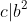. Let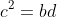, we have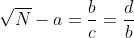where 0 < d < b, which is a contradiction.

Now a interesting problem arises: why the contradictory result from "simpliest fraction" shows that the statement is false?
You may say that axioms of arithmetics are parallel, therefore if A is equivalent to B and B is proved to be false, then A is false as well.
Meanwhile we can say the lack of simpliest solution led to the infinite descent of solution set which is eventually out of our range [of concern], and therefore such case would not happen and the statement is false.

Similar arguement can be make to prove the irrationality of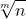.
Theorem.is either integer or irrational.
Proof. Exercise.

Now we extend numbers to algebraic numbers.
Rational Root Theorem For a polynomial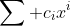with integral coefficients, rational roots, if exist, in forms of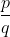, then p and q must be integral factor of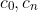respectively.
Proof. Exercise. (It's trivial when the rational numberis directly subbed into the polynomial. Otherwise Gauss' Lamma can be used.

As a special case of it, we have
Theorem. For polynomial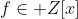with integral coefficient 1, its real root is either integer or irrational.

Example.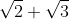is irrational.
Let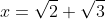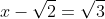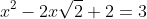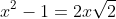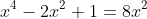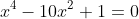is the original equation with root. Quickly check that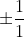is not a root of it, and therefore this polynomial has no integral zeros, so its roots so as the concerned number, must be irrational.

It provides us a powerful test for reducibility of polynomials or to test the existance of integral roots, in which we will discuss next time including Eisenstein's irreducible criterion and more tests on irrational numbers by infinite series.